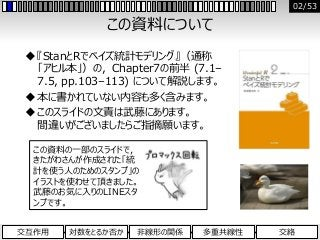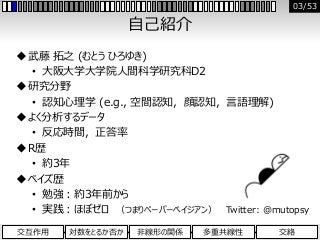Successfully reported this slideshow.×
1 of 53

Osaka.Stan #4 Chapter 7 回帰分析の悩みどころ (7.1–7.5)

31

Share

『StanとRでベイズ統計モデリング』読書会 (Osaka.Stan #4 2017.4.29）の発表資料です。Chapter 7の前半 (7.1-7.5) の内容（交互作用・対数変換・非線形回帰・多重共線性・交絡）について解説しています。
12/9追記：このスライドの紹介記事をブログに投稿しました。コピペしやすいRとStanのコードも載せています。
URL: http://bayesmax.sblo.jp/article/181782207.html

See all

Osaka.Stan #4 Chapter 7 回帰分析の悩みどころ (7.1–7.5)

1. 1. Chapter 7 (7.1–7.5) 回帰分析の悩みどころ (前半) 『StanとRでベイズ統計モデリング』読書会 (Osaka.Stan #4) 2017年4月29日 大阪大学大学院人間科学研究科D2・日本学術振興会 武藤 拓之 (Hiroyuki Muto) 01/53 交互作用 対数をとるか否か 非線形の関係 多重共線性 交絡
2. 2. この資料について 『StanとRでベイズ統計モデリング』（通称 「アヒル本」）の，Chapter7の前半 (7.1– 7.5, pp.103–113) について解説します。 本に書かれていない内容も多く含みます。 このスライドの文責は武藤にあります。 間違いがございましたらご指摘願います。 この資料の一部のスライドで， きたがわさんが作成された「統 計を使う人のためのスタンプ」の イラストを使わせて頂きました。 武藤のお気に入りのLINEスタ ンプです。 02/53 交互作用 対数をとるか否か 非線形の関係 多重共線性 交絡
3. 3. 自己紹介 武藤 拓之 (むとう ひろゆき) • 大阪大学大学院人間科学研究科D2 研究分野 • 認知心理学 (e.g., 空間認知，顔認知，言語理解) よく分析するデータ • 反応時間，正答率 R歴 • 約3年 ベイズ歴 • 勉強：約3年前から • 実践：ほぼゼロ （つまりペーパーベイジアン） Twitter: @mutopsy 03/53 交互作用 対数をとるか否か 非線形の関係 多重共線性 交絡
4. 4. 7.1 交互作用 交互作用 対数をとるか否か 非線形の関係 多重共線性 交絡 04/53
5. 5. 交互作用とは 交互作用 (interaction) • ある説明変数の効果が， 別の説明変数の値によって変化すること。 • モデル式では， 「回帰分析において説明変数同士の掛け算の項を考慮す ること (p.103)」 ※掛け算でなくても良いが，掛け算が最も頻繁に使われる。 交互作用の例 • ある薬は成人には有効だが未成年には害をなす。 • 真珠を人にあげると喜ばれるが，豚にあげても喜ばれない。 • 「※ただしイケメンに限る」 (中西, 2013) 交互作用 対数をとるか否か 非線形の関係 多重共線性 交絡 05/53 中西 大輔 [daihiko] (2013年7月15日). 「ただしイケメンに限る」ってのは要するに交互作用のことだから、交互作用の説 明をする時に例に出すと分かりやすいね。[ツイート]. Retrieved from <https://twitter.com/daihiko/status/356980280411684864>.
6. 6. 交互作用項を含まないモデル 男性の「魅力」を「容姿」と「年収」で説明するモデル  魅力：1 (低魅力)から10 (高魅力) の10段階評価  容姿：1 (イケメン) か0 (非イケメン) かの2値変数  年収：単位は万 架空データを，交互作用項を含まないモデルで分析してみる。 まずはlm関数で分析してみる。（Stanコードも後述） μ[n] = b1 + b2Ikemen[n] + b3Nenshu[n] n = 1, …, N Y[n] ~ Normal (μ[n], σ) n = 1, …, N ※清水先生の以下の記事に書かれている例を参考にしました。 重回帰分析で交互作用を検討する<http://norimune.net/1733> 交互作用 対数をとるか否か 非線形の関係 多重共線性 交絡 06/53
7. 7. 交互作用項を含まないモデル_結果 lm()の結果：  年収が100万円上がると平均魅力が0.44上がる。  イケメンは非イケメンよりも平均魅力が5.35高い。 この解釈は妥当？  年収の効果は容姿とは無関係に認められるのか？  「年収の高いイケメンは年収の低いイケメンよりもモテる」 と言える？ 交互作用 対数をとるか否か 非線形の関係 多重共線性 交絡 07/53
8. 8. 交互作用項を含むモデル 先ほどのモデルに交互作用項を追加。 この式は次のように変形できる。 μ[n] = b1 + b2Ikemen[n] + b3Nenshu[n] + b4Ikemen[n]Nenshu[n] n = 1, …, N Y[n] ~ Normal (μ[n], σ) n = 1, …, N μ[n] = b1 + b2Ikemen[n] + (b3 + b4Ikemen[n])Nenshu[n] n = 1, …, N Y[n] ~ Normal (μ[n], σ) n = 1, …, N 年収の効果は，傾きb3だけでなく， イケメンか否かによって変わる，という仮定。 交互作用 対数をとるか否か 非線形の関係 多重共線性 交絡 08/53
9. 9. 交互作用項を含むモデル_結果 lm()の結果：  交互作用が有意 ＝年収が魅力に与える影響はイケメンかどうかで変わる。 交互作用 対数をとるか否か 非線形の関係 多重共線性 交絡 09/53
10. 10. 交互作用項を含むモデル_解釈 恋愛はお金じゃない。※ただしイケメンに限る ※あくまで架空のデータ上での話です。 データを眺めてみる。 イケメン： 年収に関係なく 魅力が高い 非イケメン： 年収が高いほど 魅力も高い イケメン 非イケメン ※交互作用の解釈を より定量的に行う方法は後述。 交互作用 対数をとるか否か 非線形の関係 多重共線性 交絡 10/53
11. 11. Stanでやってみる_交互作用なしモデル 交互作用項を含まないモデル（Chapter 5の復習） data { int N; int<lower=1, upper=10> Miryoku[N]; int<lower=0, upper=1> Ikemen[N]; real<lower=0> Nenshu[N]; } parameters{ real b; real<lower=0> sigma; } model{ for (n in 1:N){ Miryoku[n] ~ normal(b + b*Ikemen[n] + b*Nenshu[n], sigma); } } 交互作用 対数をとるか否か 非線形の関係 多重共線性 交絡 11/53
12. 12. lm()とStanの比較_交互作用なしモデル lm()の結果： Stanの結果： μ[n] = b1 + b2Ikemen[n] + b3Nenshu[n] n = 1, …, N Y[n] ~ Normal (μ[n], σ) n = 1, …, N 同様の結果。 交互作用 対数をとるか否か 非線形の関係 多重共線性 交絡 12/53
13. 13. Stanでやってみる_交互作用ありモデル 交互作用項を含むモデル（青字の部分が相違点） data { int N; int<lower=1, upper=10> Miryoku[N]; int<lower=0, upper=1> Ikemen[N]; real<lower=0> Nenshu[N]; } parameters{ real b; real<lower=0> sigma; } model{ for (n in 1:N){ Miryoku[n] ~ normal(b + b*Ikemen[n] + b*Nenshu[n] + b*Ikemen[n]*Nenshu[n], sigma); } } 交互作用 対数をとるか否か 非線形の関係 多重共線性 交絡 交互作用項 (2つ の説明変数の積) をモデルに追加 13/53
14. 14. lm()とStanの比較_交互作用ありモデル lm()の結果： Stanの結果： 同様の結果。 μ[n] = b1 + b2Ikemen[n] + b3Nenshu[n] + b4Ikemen[n]Nenshu[n] n = 1, …, N Y[n] ~ Normal (μ[n], σ) n = 1, …, N 交互作用 対数をとるか否か 非線形の関係 多重共線性 交絡 14/53
15. 15. 交互作用を解釈するために このモデル式から次のことが言える：  b1 ：Ikemen = 0の時の 年収の切片  b3 ： 年収の傾き  b1 + b2 ：Ikemen = 1の時の 年収の切片  b3 + b4 ： 年収の傾き Stanでこれらの値を推定してみる。 μ[n] = b1 + b2Ikemen[n] + b3Nenshu[n] + b4Ikemen[n]Nenshu[n] = (b2 + b4 Nenshu[n])Ikemen[n]+ b1 + b3Nenshu[n] Ikemen = 0の時に0になる部分 交互作用 対数をとるか否か 非線形の関係 多重共線性 交絡 15/53
16. 16. Stanでパラメータの和を推定_モデル transformed parametersブロックを追加 data {（省略）} parameters{（省略）} transformed parameters{ real Intrcpt_I0; real Slope_I0; real Intrcpt_I1; real Slope_I1; Intrcpt_I0 = b; Slope_I0 = b; Intrcpt_I1 = b + b; Slope_I1 = b + b; } model{（省略）} ※分かりやすさを優先してあえて冗長に書いています。 交互作用 対数をとるか否か 非線形の関係 多重共線性 交絡 16/53
17. 17. Stanでパラメータの和を推定_結果 年収が魅力に与える効果（切片と傾き）を， イケメンの場合と非イケメンの場合とに分けて推定できた。 さらにtransformed parameterを追加すれば， 「イケメンと非イケメンで傾きの差がどのくらいか」等も推定できる。 交互作用 対数をとるか否か 非線形の関係 多重共線性 交絡 17/53
18. 18. ダミー変数を用いた交互作用項 3水準以上から成るカテゴリカル変数を 説明変数とするときの交互作用項を考える  例えば，学生時代の所属クラブ。 カテゴリーの数だけ，2値のダミー変数を作成。 (コーディングの方法によっては「カテゴリー数 – 1」個のダミー変数で十分。) 特定のカテゴリに所属している人数が少ないと 推定がうまくいかない。 →ゆるい制約を含めた階層モデルを使う (8章) (b3 + b4 C1[n] + b5 C2[n] + b6 C3[n] + b7 C4[n] )Nenshu [n] Cj[n] = 0 (nがjに所属していないとき) 1 (nがjに所属しているとき) 交互作用 対数をとるか否か 非線形の関係 多重共線性 交絡 18/53
19. 19. 交互作用項を含めるか否か 交互作用の悩みどころ  解釈が難しくなりがち。 特に，連続変量同士の交互作用や 3つ以上の変数間の交互作用を見る場合。  説明変数が多いと組み合わせが膨大になる。 どんな時に交互作用項を入れるか  交互作用そのものに関心がある場合 （交互作用が「ない（小さい）」ことを主張したい場合を含む）  データを眺めて明らかに交互作用が確認できる場合  背景知識から交互作用の存在が予想できる場合 交互作用 対数をとるか否か 非線形の関係 多重共線性 交絡 19/53
20. 20. 7.2 対数をとるか否か 交互作用 対数をとるか否か 非線形の関係 多重共線性 交絡 20/53
21. 21. 変数変換の例 ローデータの値を変換して分析に用いることがある。 （例）  線形変換  対数変換（非線形変換）  逆数変換（非線形変換） X’[n] = aX[n] + b n = 1, …, N X’[n] = 1/X[n] n = 1, …, N X’[n] = logaX[n] n = 1, …, N 交互作用 対数をとるか否か 非線形の関係 多重共線性 交絡 21/53
22. 22. 非線形変換による分布の変化 データの分布を正規分布に近づけるために 対数変換や逆数変換が行われることがある。 変数変換すると解釈が難しくなる場合が多い。 交互作用 対数をとるか否か 非線形の関係 多重共線性 交絡 X = {53.05, 63.76, …} logX = {3.97, 4.16, …} 1/X = {.019, .016, …} 非線形変換の例： 22/53
23. 23. 非線形変換の影響の例 (大久保, 2011) 1. 元の変数が持つ差や比の情報が失われる。 1. ローデータには存在しなかった「差の差」や「差の比」が検出される。 X1 X2 X2−X1 Y1 Y2 Y2−Y1 Xn 1.000 2.000 1.000 11.000 12.000 1.000 logXn 0.000 0.301 0.301 1.041 1.079 0.038 1/Xn 1.000 0.500 −0.500 0.091 0.083 −0.008 |(X2−X1)−(Y2−Y1)| (X2−X1)/(Y2−Y1) Xn 0.000 1.000 logXn 0.263 7.920 1/Xn 0.492 62.500 値が大きいほど差が過小評価 交互作用項の推定結果が歪む危険性 大久保 街亜 (2011). 反応時間分析における外れ値の処理 専修人間科学論集心理学篇 1, 81-89. 交互作用 対数をとるか否か 非線形の関係 多重共線性 交絡 23/53
24. 24. 対数変換が有用なケース(1) 「Aが〇〇倍になるとBが××倍になる」と仮定（解釈）したいとき 1. 説明変数と応答変数に元の値を使ったモデル →X[n]がk増えるとY[n]の平均値がkb2だけ増える。 1. 説明変数と応答変数を対数変換したモデル →X[n]がk倍になるとY[n]の最頻値が{10^b2log10(k)}倍になる。 ※Y[n]は対数正規分布に従うためY[n]の平均値は最頻値と一致しない。 log10(Y[n]) = b1 + b2log10(X[n]) + e n = 1, …, N e ~ Normal (0, σ) Y[n] = b1 + b2X[n] + e n = 1, …, N e ~ Normal (0, σ) 交互作用 対数をとるか否か 非線形の関係 多重共線性 交絡 24/53
25. 25. 参考：なぜ「〇〇倍」と解釈できるかの説明 元のモデル式は， log10(Y[n]) = b1 + b2log10(X[n]) + e n = 1, …, N ここで，X[n] = aの時とX[n] = kaの時， log10(𝑦0) = b1 + b2log10(a) ･･････① log10(𝑦1) = b1 + b2log10(ka) ･･････② ②を変形して， log10(𝑦1) = b1 + b2log10(a) + b2log10(k) = log10(𝑦0) + b2log10(k) (∵①) したがって，𝑦1の最頻値 ෝ𝑦1は， ෝ𝑦1 = 10^{log10( ෝ𝑦0) + b2log10(k)} = 10^log10( ෝ𝑦0) × 10^b2log10(k) = ෝ𝑦0× 10^b2log10(k) (∵②) ∴X[n]がk倍になるとX[n]の最頻値は{10^b2log10(k)} 倍になる。 交互作用 対数をとるか否か 非線形の関係 多重共線性 交絡 25/53
26. 26. 交互作用 対数をとるか否か 非線形の関係 多重共線性 交絡 賃貸データで結果の比較 (1) 元のデータの散布図と予測分布 対数変換したデータの散布図と予測分布 薄い灰帯：80%予測区間 濃い灰帯：50%予測区間 黒線：中央値 予測区間に負の値を含む これらの値に 結果が引きずられる （頑健性が低い） 26/53
27. 27. 交互作用 対数をとるか否か 非線形の関係 多重共線性 交絡 賃貸データで結果の比較 (2) 元のデータの実測値と予測値のプロットおよびノイズの分布 対数変換したデータの実測値と予測値のプロットおよびノイズの分布 予測区間に負の値を含む エラーバーは80%予測区間 予測区間が広い 正規分布と乖離 27/53
28. 28. 対数変換が有用なケース(2) 倍々で増える仕組みが想定される場合 e.g., 複利，がん細胞の分裂 例えば次のようなモデルを想定： (e.g., 時間がXだけ経過した後の細胞の数) このままだとb1もb2も解釈しづらい。 説明変数と応答変数を対数変換 (底は2) すると…… ＝X[n]が1増えるとY[n]の最頻値が(2^b2)倍になる Y[n] = b1 + b2×2^X[n] + e n = 1, …, N log2(Y[n]) = b1 + b2×2^log2X[n] + e n = 1, …, N = b1 + b2X[n] + e 交互作用 対数をとるか否か 非線形の関係 多重共線性 交絡 28/53
29. 29. 変数変換のまとめ 解釈が難しくなる場合には変数変換は避けるのがベター。  むりやり正規分布に近づけるのではなく， データの分布に適したモデルを選択する。 対数変換したほうが解釈しやすい場合もある。  「Aが〇〇倍になるとBが××倍になる」と仮定したいとき  倍々で増える仕組みが想定されるとき 解釈しやすいモデルが「正しい」とは限らない。  あくまでもその仮定を選んだというだけ。(Chapter 3, p.22参照) 変数変換とモデリングは表裏一体？  モデルに合わせて変数変換するのではなく， 変数変換のメカニズムを含むモデルを構築できる。 交互作用 対数をとるか否か 非線形の関係 多重共線性 交絡 29/53
30. 30. 7.3 非線形の関係 交互作用 対数をとるか否か 非線形の関係 多重共線性 交絡 30/53
31. 31. 線形/非線形な相関関係 いろいろな相関関係 (rはピアソンの積率相関係数=線形の関係性の強さ) よくある線形モデルではうまく説明できない相関関係もある。 非線形な関連性へのアプローチ： 1. 非線形を生み出すメカニズムをモデルに反映 2. シンプルで解釈しやすい曲線の当てはめ (e.g., n次多項式曲線，三角関数の曲線) r = .71 r = −.03 r = −.04 r = .04 交互作用 対数をとるか否か 非線形の関係 多重共線性 交絡 31/53
32. 32. 非線形な関係の例 スポーツ場面における緊張感とパフォーマンスの関係 薬剤投与からの経過時間と血中濃度の関係 緊張の程度 パフォーマンス 高い 低い リラックス 緊張 交互作用 対数をとるか否か 非線形の関係 多重共線性 交絡 32/53
33. 33. 交互作用 対数をとるか否か 非線形の関係 多重共線性 交絡 二次曲線の当てはめ_モデル data { int N; real X[N]; real Y[N]; } parameters{ real a; real b; real x0; real<lower=0> s_Y; } model{ for (n in 1:N){ Y[n] ~ normal(a + b*(X[n]-x0)^2, s_Y); } } μ[n] = a + b(X[n]−x0)2 n = 1, …, N Y[n] ~ Normal (μ[n], σ) n = 1, …, N 事前知識を用いて適宜制約を課す。例えば二次 曲線が上に凸であることが事前知識から明らかな場 合にはbの上限を0とする。制約がないと収束しない 場合があるので注意。 33/53
34. 34. 二次曲線の当てはめ_結果 緊張の程度 パフォーマンス 高い 低い リラックス 緊張 μ[n] = a + b(X[n]−x0)2 n = 1, …, N Y[n] ~ Normal (μ[n], σ) n = 1, …, N 交互作用 対数をとるか否か 非線形の関係 多重共線性 交絡 34/53
35. 35. 時系列データへの指数曲線の当てはめ_モデル data { int N; real X[N]; real Y[N]; } parameters { real<lower=0, upper=100> a; real<lower=0, upper=5> b; real<lower=0> s_Y; } model { for (n in 1:N) Y[n] ~ normal(a*(1 - exp(-b*X[n])), s_Y); } μ[n] = a{1 − exp(− bX[n])} n = 1, …, N Y[n] ~ Normal (μ[n], σ) n = 1, …, N 事前知識を用いて適宜制約を課す。 交互作用 対数をとるか否か 非線形の関係 多重共線性 交絡 35/53
36. 36. μ[n] = a{1 − exp(− bX[n])} n = 1, …, N Y[n] ~ Normal (μ[n], σ) n = 1, …, N a：頭打ちの大きさを決めるパラメータ b：頭打ちになるまでの時間を決めるパラメータ 交互作用 対数をとるか否か 非線形の関係 多重共線性 交絡 時系列データへの指数曲線の当てはめ_結果 36/53
37. 37. パラメータに制約を課さなかった場合 収束せず data {（省略）} parameters { real a; real b; real<lower=0> s_Y; } model {（省略）} パラメータの範囲を指定しないと…… 交互作用 対数をとるか否か 非線形の関係 多重共線性 交絡 37/53
38. 38. 7.4 多重共線性 交互作用 対数をとるか否か 非線形の関係 多重共線性 交絡 38/53
39. 39. 交互作用 対数をとるか否か 非線形の関係 多重共線性 交絡 多重共線性とは  多重共線性 (multicolinearity; マルチコ) • 回帰分析において，説明変数間の相関が高いと……  回帰係数の標準誤差が大きくなる  回帰係数の符号が直感に反する結果となり， 解釈が困難となる場合がある。  回帰係数が収束せず，うまく推定できないことがある。 ※モデルによる予測に関心があり，回帰係数の解釈をしないのであれば，多重 共線性は必ずしも問題とならない。  多重共線性がある場合の例 英検のグレード TOEICスコア 年収 高い正の相関 39/53
40. 40. モデル式から見た多重共線性の説明 重回帰分析のモデル式 (説明変数：A[n] と B[n] ) 説明変数A[n]とB[n]の相関が高い時の近似式 この時，例えばb2 + b3 = 3が真の値の時， (b2, b3) の組み合わせは一意に決まらない。 e.g., (1, 2), (−5, 8), (123.45, −120.45) μ[n] = b1 + b2A[n] + b3B[n] n = 1, …, N Y[n] ~ Normal (μ[n], σ) n = 1, …, N A[n] ≅ B[n] のとき， μ[n] = b1 + (b2 + b3) A[n] n = 1, …, N Y[n] ~ Normal (μ[n], σ) n = 1, …, N 交互作用 対数をとるか否か 非線形の関係 多重共線性 交絡 40/53
41. 41. Rのlm関数でシミュレーション 多重共線性がある時とない時で 結果がどのように変わるのかをシミュレーションしてみる。 古典的な重回帰分析で検証。  説明変数はA・Bの2つとする。  ρAB (母相関係数) を変化させてみる。 μ[n] = b1 + b2A[n] + b3B[n] n = 1, …, N Y[n] ~ Normal (μ[n], σ) n = 1, …, N ※Stanで同様のシミュレーションを行ってもほぼ同じ結果が得られたため，Stanの例は 省略。今回のような簡単なモデルであれば，収束しないということはなさそう？ 交互作用 対数をとるか否か 非線形の関係 多重共線性 交絡 41/53
42. 42. Rのlm関数でシミュレーション_コード ##パラメータ等の指定## rAB <- 0.9 #説明変数間の母相関係数。この値をいろいろ変えてみる。 rAY <- 0.5 #AとYの母相関係数 rBY <- 0.6 #BとYの母相関係数 n <- 100 #サンプルサイズ Rep <- 1000 #サンプリング回数 ##分散・共分散行列の作成## Mat <- matrix(c(1, rAB, rAY, rAB, 1, rBY, rAY, rBY, 1), ncol=3) ##N = nのデータセットをRep回生成し，それぞれに対して重回帰分析## Res_beta <- data.frame(b2 = 1:n, b3 = 1:n) for (i in 1:Rep){ d <- as.data.frame(mvrnorm(n= n, mu= c(0, 0, 0), Sigma= Mat, empirical= FALSE)) colnames(d) <- c("A","B","Y") reg <- lm(Y ~ A + B, data = d) Res_beta[i,] <- reg\$coefficients[2:3] } Res_beta #Rep回分の偏回帰係数 (b2とb3) が格納されたデータフレーム。 交互作用 対数をとるか否か 非線形の関係 多重共線性 交絡 42/53
43. 43. 交互作用 対数をとるか否か 非線形の関係 多重共線性 交絡 Rのlm関数でシミュレーション_結果 ρAB= .90のとき（多重共線性あり） ρAB= .20のとき（多重共線性なし） 43/53
44. 44. 交互作用 対数をとるか否か 非線形の関係 多重共線性 交絡 Rのlm関数でシミュレーション_結果 ρAB= .90のとき（多重共線性あり） ρAB= .20のとき（多重共線性なし）多重共線性があると……  推定値のばらつきが大きい  符号が逆転傾向 e.g., TOEICスコアが高いと年収が上がり， 英検のグレードが高いと年収が下がる？ 44/53
45. 45. 多重共線性の問題を回避するには 1. 説明変数間の相関が背景知識から明らかな場合， 片方を捨てるのが簡単。  |r| > .80～.95  VIF > 10 ※VIF (variance inflation factor) = 1/(1-r2)  どの変数を捨てるかの判断は慎重に リサーチクエスチョンは何？ 興味のある情報を最も多く反映した変数はどれ？ 2. 両方の説明変数の情報を含む別のモデルを考案する。 • 合成得点の算出 • 潜在変数を仮定したモデルの使用 交互作用 対数をとるか否か 非線形の関係 多重共線性 交絡 45/53
46. 46. 参考：潜在変数を仮定したモデルの例 英検のグレード TOEICスコア 年収 A社独自の英語 テストのスコア 英語力 潜在変数 （直接観測されない変数） 交互作用 対数をとるか否か 非線形の関係 多重共線性 交絡 46/53 ※このパス図は，3種類の英語のテストから推定された英語力が年収に影響を与える モデルを表しているが，実質的には因子数1の因子分析モデルと等価である。 （年収変数の位置を右側に移動すると分かりやすい。）
47. 47. 7.5 交絡 交互作用 対数をとるか否か 非線形の関係 多重共線性 交絡 47/53
48. 48. 交互作用 対数をとるか否か 非線形の関係 多重共線性 交絡 交絡とは 交絡 (confounding) • 「モデルの外側に応答変数と説明変数の両方に影響を与 える変数が存在すること (p.112)」 • 体重が重い小学生ほど足が速い？ 体重足の速さ + 48/53
49. 49. 交互作用 対数をとるか否か 非線形の関係 多重共線性 交絡 交絡とは 交絡 (confounding) • 「モデルの外側に応答変数と説明変数の両方に影響を与 える変数が存在すること (p.112)」 • 体重が重い小学生ほど足が速い？ • 年齢が増えると体重が増える • 年齢が増えると筋力が増して足が速くなる • 体重が増えすぎると走る速さは遅くなる 体重足の速さ 年齢 隠れた変数 ++ − 49/53
50. 50. 何が交絡してる？ 発表者の手元にあるデータ • 身長が高い人ほど方向感覚が優れている。 • 背が高いとより遠くが見渡せるから？ 身長方向感覚 + 交互作用 対数をとるか否か 非線形の関係 多重共線性 交絡 50/53
51. 51. 何が交絡してる？ 発表者の手元にあるデータ • 身長が高い人ほど方向感覚が優れている。 • 背が高いとより遠くが見渡せるから？ • 男性の方が女性よりも平均身長が高い • 男性の方が女性よりも平均的に空間把握能力が高い 身長方向感覚 性別 隠れた変数 + 交互作用 対数をとるか否か 非線形の関係 多重共線性 交絡 51/53
52. 52. パス解析のすすめ パス解析 (path analysis) • 「複数の回帰を組み合わせて 変数間の因果関係を模索する解析 (p.113)」 • 交絡変数を考慮できる。 • パス図を描くと視覚的に理解しやすい。 パス解析のコツ • 解釈しやすいシンプルな仮定を優先すると良い。 （複雑なモデルは解釈しにくい） • 利用できる背景知識をモデルに組み込む。 • モデルの改善には試行錯誤が欠かせない。 体重足の速さ 年齢 交互作用 対数をとるか否か 非線形の関係 多重共線性 交絡 52/53
53. 53. そうだ ベイズ、しよう。 53/53